Disclosure: This post may contain affiliate links, meaning when you click the links and make a purchase, we receive a commission.

# How to Remove Commas in Excel [9 Smart Ways]

In this article, I will show you how to remove commas from the names (placed in the middle/in-between/last) and the numbers in Excel.

At first, download the workbook that I have used to write this tutorial.

## How do I remove commas from numbers in Excel?

In many ways, commas are added to the numbers. Let’s see how we can remove the commas from the numbers.

### 1) Converting commas to the decimal point

If you have imported some numbers from other places (for example, pdf), the numbers might have commas in the place of decimal points.

You’re seeing some text numbers in the following image.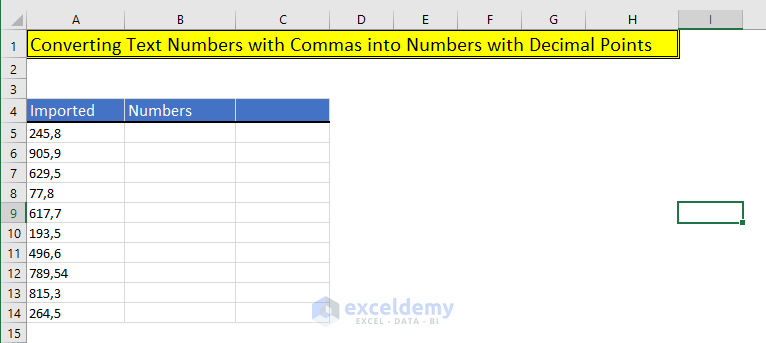The commas are actually placed instead of decimal points. So, we want to convert these text numbers replacing the commas with the decimal points.

#### Method 1: Using Excel SUBSTITUTE function

Excel’s SUBSTITUTE Function replaces the existing text with new text in a text string.

Syntax of SUBSTITUTE Function: =SUBSTITUTE(text, old_text, new_text, [instance_num])

Where,

• text (Required): The text or the cell reference where the text is that you want to change.
• old_text (Required): The text or character you want to replace.
• new_text (Required): The text that will replace the old text.
• instance_num (Required): The number of the old texts will be replaced with the new text. If you don’t use any value for this parameter, all old texts will be replaced by the new one.

Step 1

I have used this formula in the cell `B5: =SUBSTITUTE(A5,",",".")`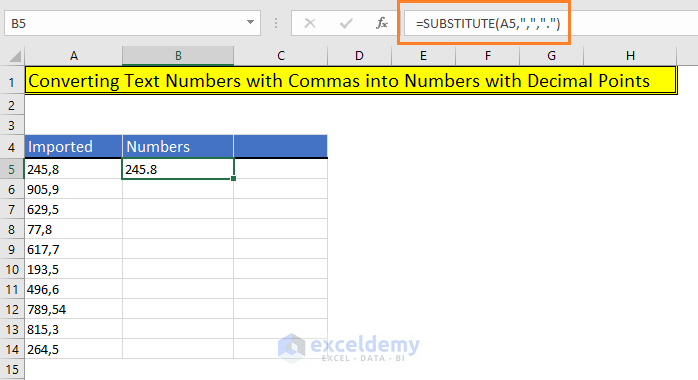You see, the return is still text, not a number (aligned left the cell).

Step 2:

To convert the text to number, we just add 0 with the formula: `B5: =SUBSTITUTE(A5,",",".") + 0`

Now, the text is converted to a number (placed right side of the cell).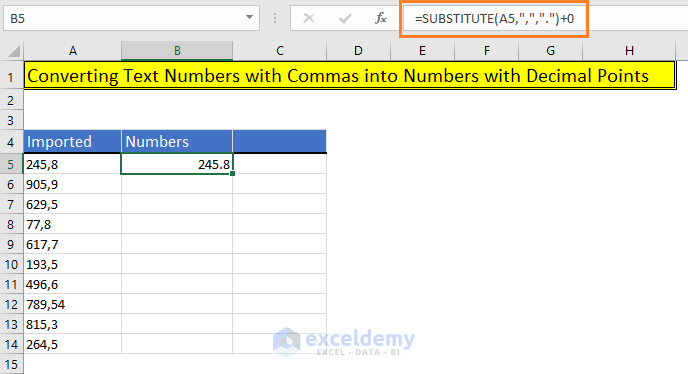Step 3:

If I copy the formula for other cells in the column, we get all the texts with commas are converted to numbers with decimal points.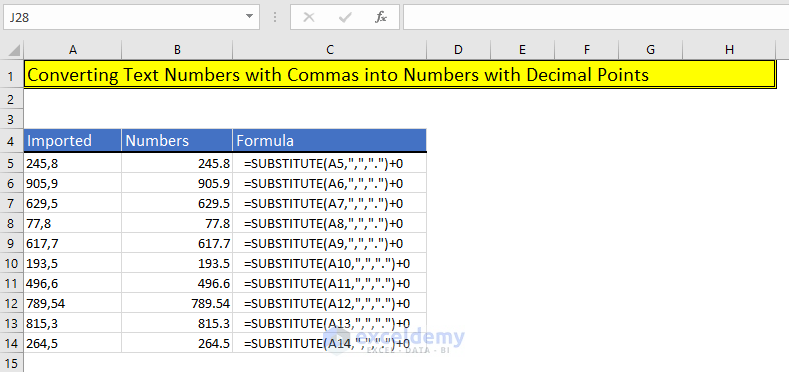#### Method 2: Using Text to Columns Wizard

We can also use Excel’s Text to Columns wizard to replace commas with decimal points.

Step 1:

Select the data where you want to apply the Text to Columns feature.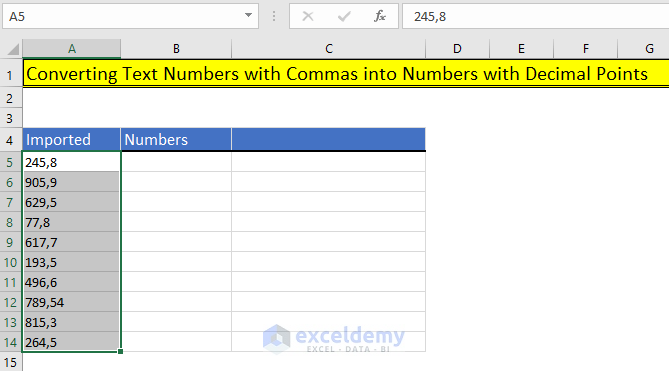Step 2:

Go to Data tab > Data Tools group of commands > Click on the Text to Columns command

Convert Text to Columns Wizard appears > Choose the Fixed width radio button > Then click on the Next button.

Keyboard shortcut to open the Convert Text to Columns Wizard: ALT + A + E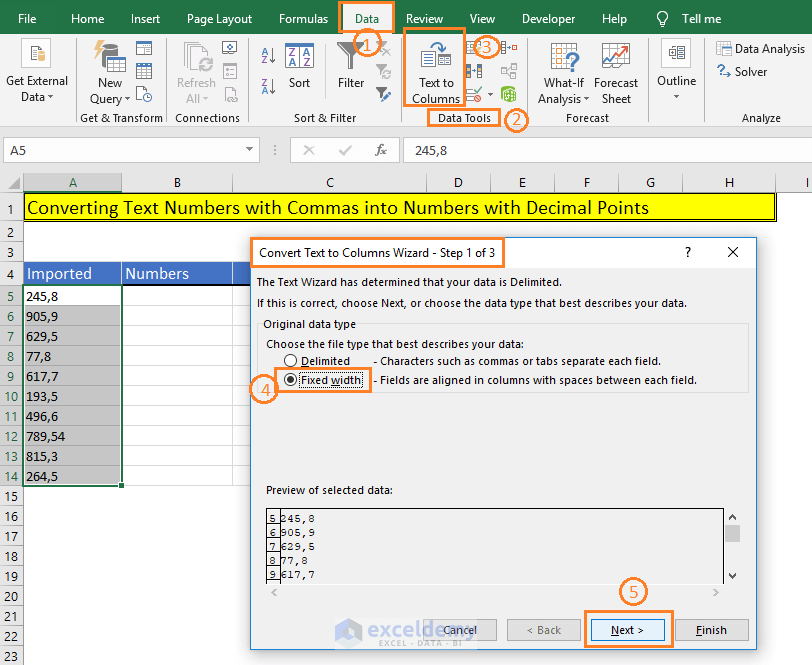Step 3:

There is nothing to do with Step 2 of 3. So, click on the Next button again.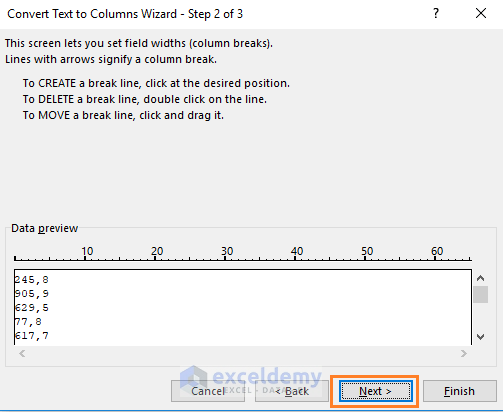Step 4:

In step 3 of 3, I click on the Advanced command > Advanced Text Import Settings dialog appears > Here there are two fields:

• Decimal separator: As the decimal separator, I choose the comma.
• And Thousands separator: I don’t change anything here.

Finally, click on the OK button.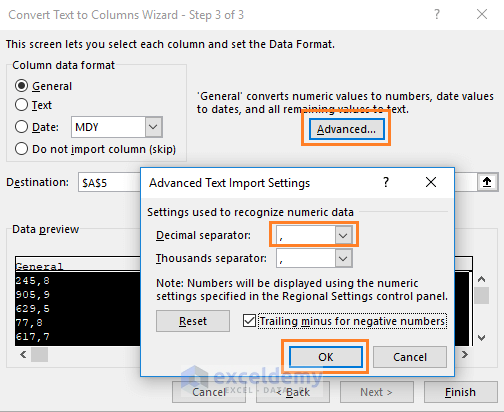Step 5:

As the destination, I select the cell B5, and then I choose the Finish button.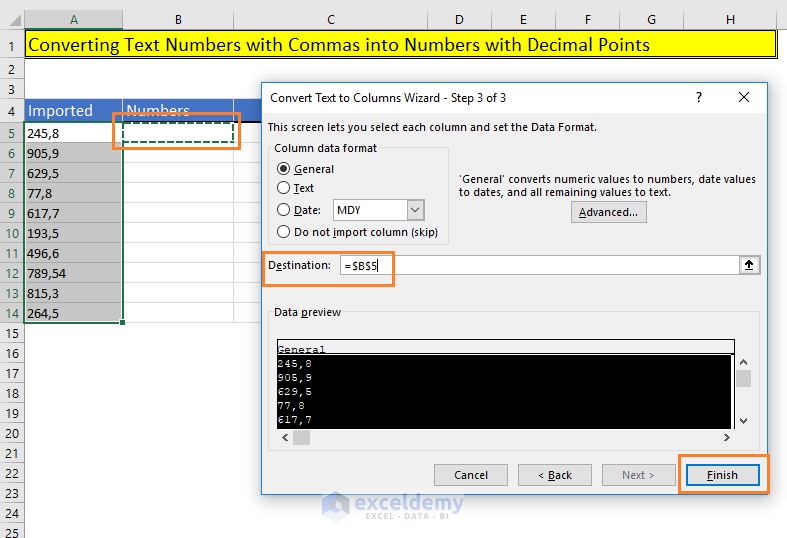This is the result I get: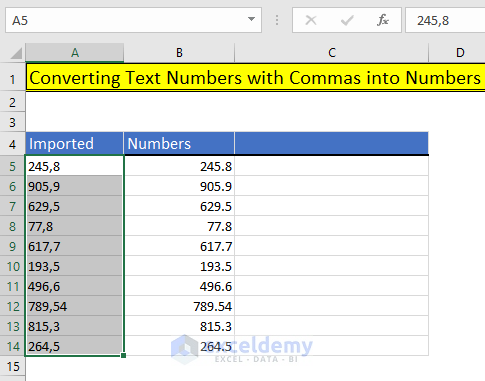You see, all the imported texts with commas are converted to numbers with decimal points.

The disadvantage of using this method: If you change the texts, the numbers will not change. So, it is better to use the formula (above method).

#### Method 3: Using Excel Replace Feature

Step 1:

Select the worksheet where the data is. Now open Excel’s Find and Replace feature. You can open Find and Replace in two ways:

• Home > Editing > Find & Select dropdown > Click on the Replace command
• Or use the keyboard shortcut: CTRL + H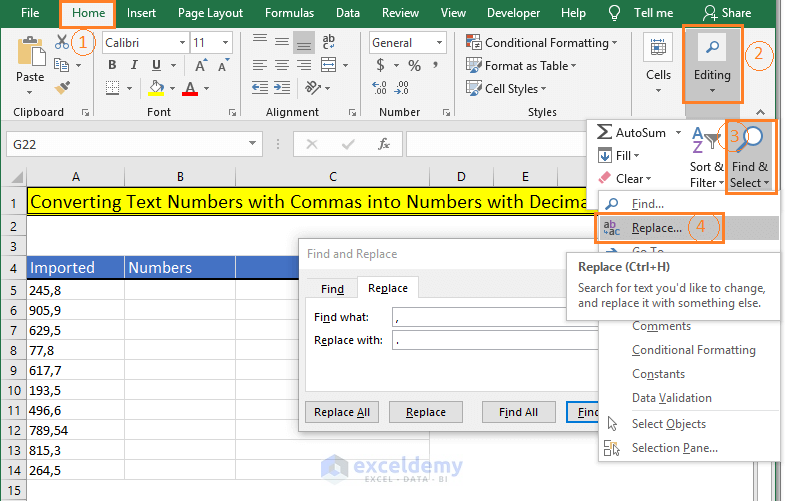In the Find and Replace, in the Find what field, input comma (,) and in the Replace with field, input decimal point (.).

And finally, click on the Replace All command.

This is what we get:

Excel shows me a message: “All done. We made 10 replacements.”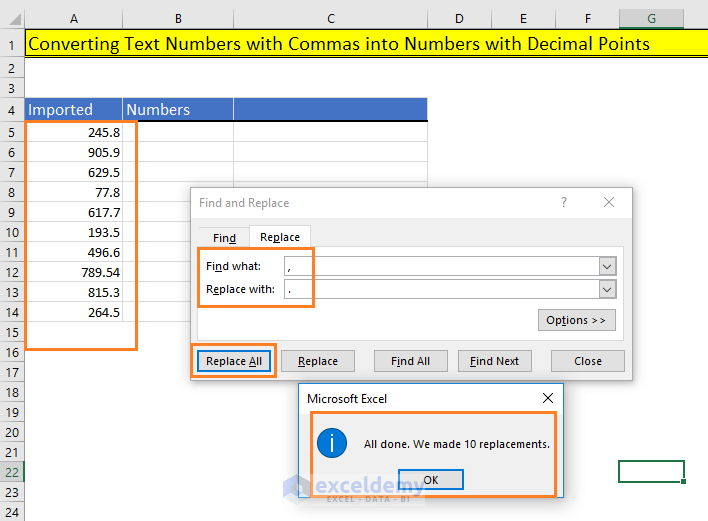You see, where the texts were, all of them are replaced with numbers with decimal points.

The disadvantage of using this method: Replacements are done in the same places.

### 2) Removing numbers after commas

In some cases, you might want to keep the number parts before the comma and want to delete the comma and the numbers after the commas.

In this case, you can use both the Text to Columns feature and an Excel formula.

#### Method 1: Using Excel Formula

In the cell B5, I have used this formula: `=LEFT(A5,SEARCH(",",A5)-1)+0`

Then copy down the formula for other cells in the column. You’re seeing the whole process in the image below.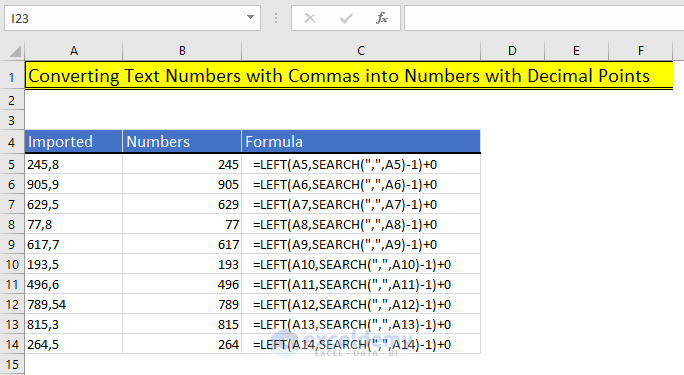How does this formula work?

`=LEFT(A5,SEARCH(",",A5)-1)+0`

Let me explain how this formula actually works:

• `SEARCH("`,”,A5)-1`)`: The Search function returns the position of the comma (,) in the text in the cell A5. The position is 4.
• Using the LEFT function, we are just returning the first 3 characters from the text.
• At the end of the formula, we are adding 0 to make the return value to a number.

#### Method 2: Using Text to Columns Wizard

Step 1:

Select the text with commas and open the Text to Columns Wizard (Data > Data Tools > Text to Columns).

Delimited is by default selected > Click on the Next button.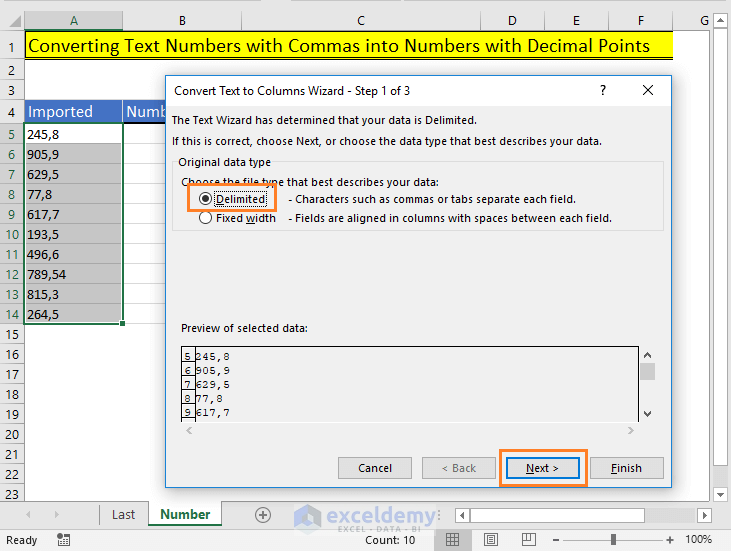Step 2:

In Step 2 of 3, select Comma delimiter (by default it is selected comma for this text set).

Just click on the Next button.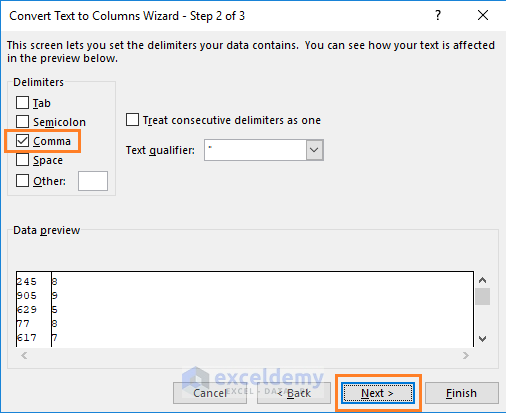Step 3:

In Step 3 of 3, I determine the Destination cell B5 and click on the Finish button.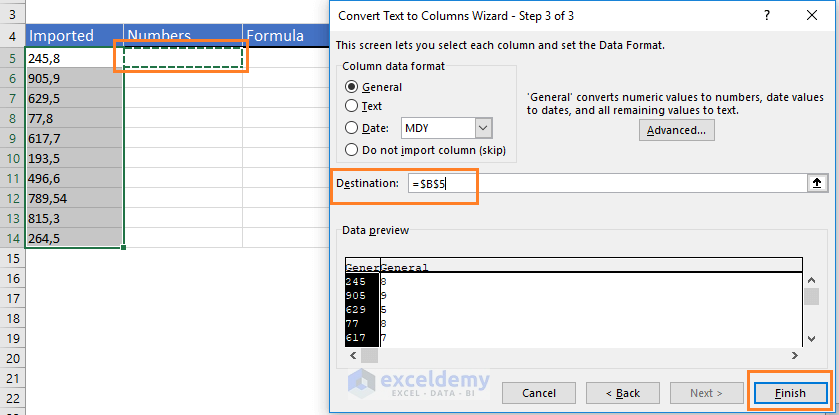This is what we get: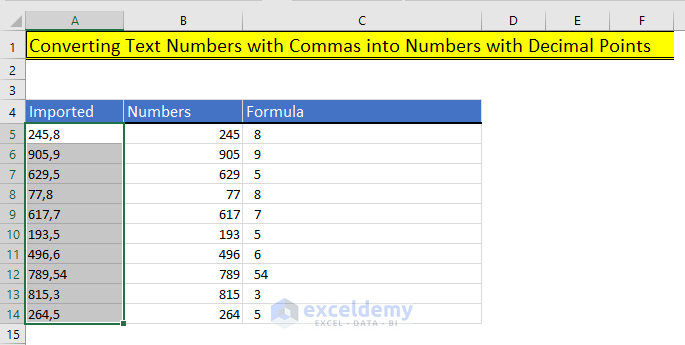The text values are divided into two columns of numbers. Commas are removed.

### 3) Removing “thousands comma separators” from the numbers

You’re seeing some numbers here. These numbers are comma-separated.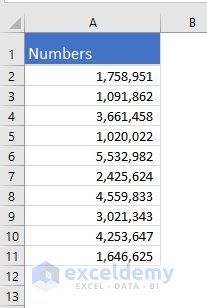To remove the commas from these numbers, you can use two methods:

#### Method 1: Applying General Format

Select the numbers > Home > Number group of commands > From the drop-down, choose the General format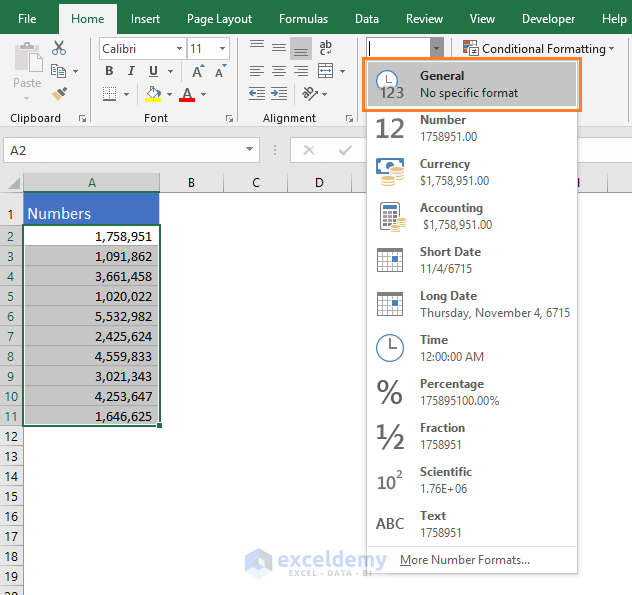This is what we get: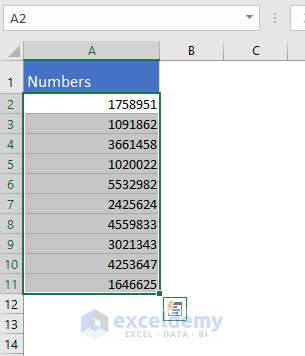#### Method 2: Using the Format Cells dialog box

We can also use the Format Cells dialog box to remove the thousand-separator commas from the numbers.

Step 1:

Select the numbers > Choose the little arrow at the bottom right corner of the Number group of commands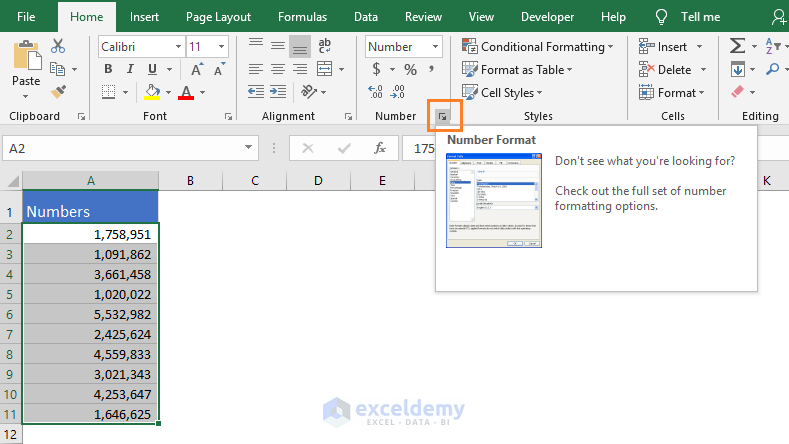Step 2:

Format Cells dialog box appears with the Number tab and Number category activated > Just uncheck the Use 1000 Separator (,) checkbox.

Finally, click on the OK button.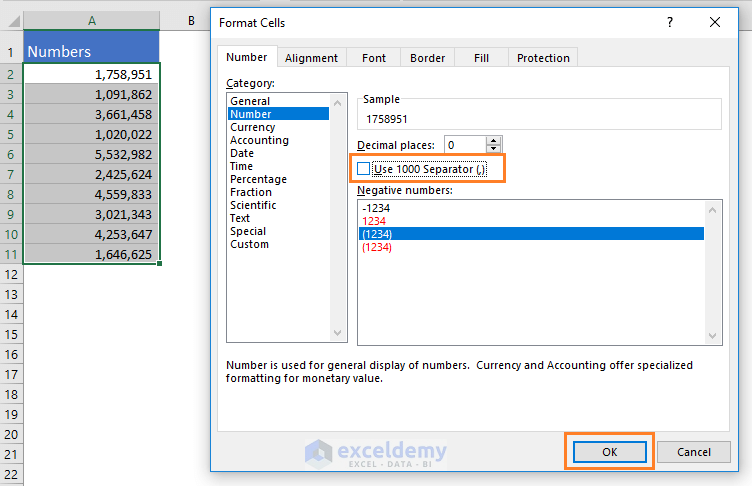Keyboard shortcut to open the Format Cells dialog box: CTRL + 1

This is what we get: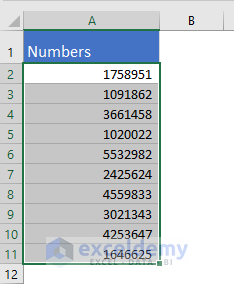## How to remove comma between names in Excel (using formula)

You’re having some names like the following image. I have also shown the desired output.

How can we do this?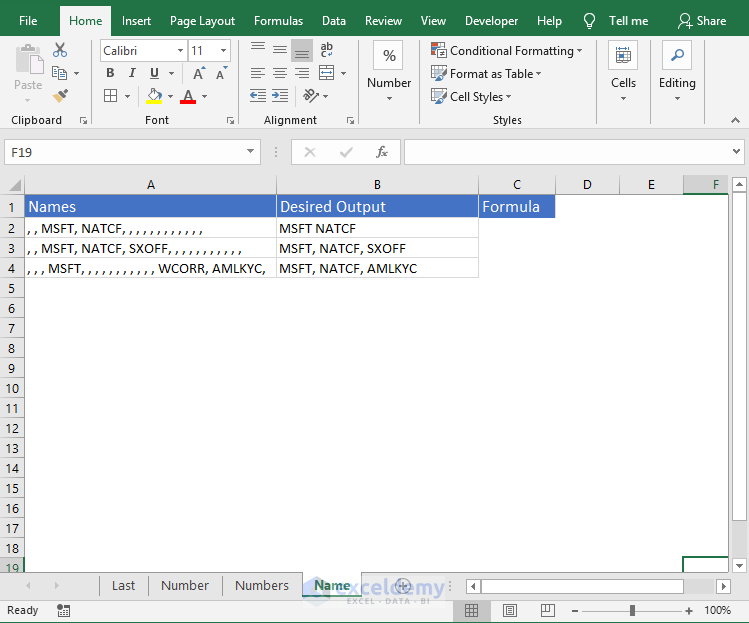I have used this formula in the cell `C2: =SUBSTITUTE(TRIM(SUBSTITUTE(A2,",",""))," ",", ")`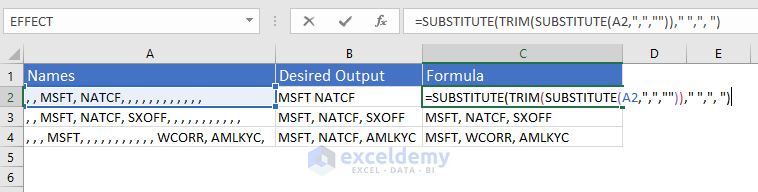How does this formula work?

• `SUBSTITUTE(A2,",","")`: This part of the formula replaces all the commas with nothing. So, it converts this text `", , MSFT, NATCF, , , , , , , , , , , ,"` to `" MSFT NATCF           "`.
• Then we apply the TRIM function to this value: `TRIM(" MSFT NATCF           ")`. It returns: `"MSFT NATCF"`
• Now we apply again SUBSTITUTE function on this returned value:

`=SUBSTITUTE("MSFT NATCF"," ",", ")`. This part replaces the Spaces with a comma and Space. The final returned value is: `="MSFT, NATCF"`

This is what we get in the cell C2.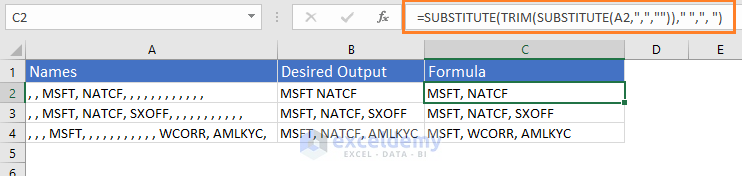## How to remove the last comma in Excel (using formula)

Suppose, you have a name and the name has a comma in the last. How will you remove that comma?

Obviously, you can use the Replace feature of Excel. Here I am showing the formula method. The whole process is shown below: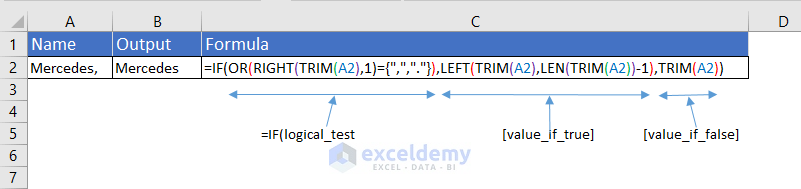I have used this formula in the cell `B2: =IF(OR(RIGHT(TRIM(A2),1)={",","."}),LEFT(TRIM(A2),LEN(TRIM(A2))-1),TRIM(A2))`

How does this formula work?

• logical_test of the IF Function: `OR(RIGHT(TRIM(A2),1)={",","."})`. Let’s analyze this part. At first TRIM function (TRIM(A2)) removes all extra spaces from the text. Then RIGHT part of the formula, RIGHT(returned_text_by_TRIM,1), returns the rightmost character from the trimmed text. Finally, OR part of the formula, `OR(right_most_character_of_trimmed_text={",","."})`, returns TRUE if the rightmost character is a comma or a period. Returns FALSE, if the rightmost character is not a comma or a period. For our value “Mercedes, “, it returns TRUE.
• value_if_true of the IF Function: LEFT(TRIM(A2),LEN(TRIM(A2))-1)

I can simplify this formula in this way: LEFT(trimmed_text,length_of_the_trimmed_text-1). So, it returns the whole trimmed text except for the last character.

• value_if_false of the IF Function: TRIM(A2)

It returns the TRIMMED Text.